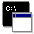## Firefly and PC GAMESS-related discussion clubLearn how to ask questions correctly

Re^4: excited state geometry opt and intersystem crossing

Jonas Baltrusaitis
jonas-baltrusaitis@uiowa.edu

My related question is:
I am running geometry optimization (thanks to Alex for input) as:

\$contrl scftyp=rhf icut=10 inttyp=hondo CITYP=CIS runtyp=OPTIMIZE nzvar=1 \$end
\$CIS nstate=4 istate=-1 MULT=1 icutcp=12 ndavit=100 MXVEC=100 \$end

the problem is that at some point I run into SCF problems with, for example

SOSCF IS SCALING ROTATION ANGLE MATRIX, DXMAX= Ā Ā3.294684

and I run out of SCF cycles. I could increase number of cycles but I am not sure if that would solve the problem as SCF convergence becomes very slow. I attached the problematic output, would appreciate comments

JOnasThis message contains the 1822 kb attachment [ output.out ] SCF fails to convergeThu Jun 10 '10 5:25pmThis message read 767 times